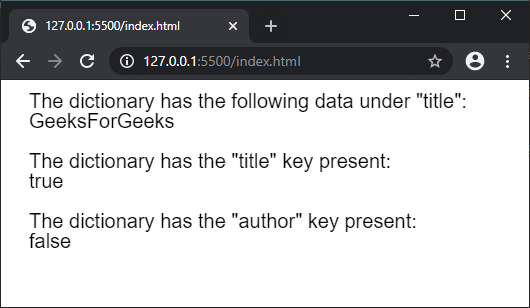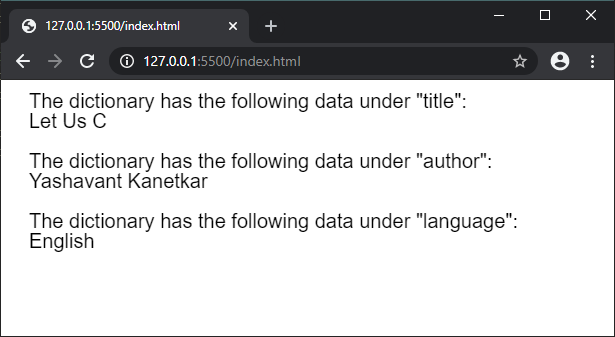GFG App
Open AppBrowser
Continue

# p5.js | createStringDict() Function

The createStringDict() function is used to create a p5.StringDict instance with the given data. The data can be individually passed as a key-value pair or given as a collection of values using an object.

Syntax:

`createStringDict(key, value)`

or

`createStringDict(object)`

Parameters:

• key: It specifies the string that is used as the key in the dictionary.
• value: It specifies the string that is used as the value in the dictionary.
• object: It specifies the object that is used as the dictionary.

Return Value: It returns a p5.StringDict object with the given data.
The program below illustrate the createStringDict() function in p5.js:
Example 1:

## javascript

 `function` `setup() {` `  ``createCanvas(500, 200);` `  ``textSize(20);`   `  ``// Creating a string dictionary` `  ``// with the given key and value pair` `  ``let mydict = createStringDict(``"title"``, ``"GeeksForGeeks"``);`   `  ``// Accessing the data using the data property` `  ``text(``'The dictionary has the following data under "title":'``, 20, 20);` `  ``text(mydict.data.title, 20, 40);`   `  ``// Checking if a key exists in the dictionary` `  ``text(``'The dictionary has the "title" key present:'``, 20, 80);` `  ``text(mydict.hasKey(``"title"``), 20, 100);`   `  ``text(``'The dictionary has the "author" key present:'``, 20, 140);` `  ``text(mydict.hasKey(``"author"``), 20, 160);` `}`

Output:Example 2:

## javascript

 `function` `setup() {` `  ``createCanvas(600, 200);` `  ``textSize(20);`   `  ``let obj = {` `    ``book: ``"Let Us C"``,` `    ``author: ``"Yashavant Kanetkar"``,` `    ``language: ``"English"` `  ``}`   `  ``// Creating a string dictionary` `  ``// with the above object` `  ``let mydict = createStringDict(obj);`   `  ``// Accessing the data using the data property` `  ``text(``'The dictionary has the following data under "title":'``, 20, 20);` `  ``text(mydict.data.book, 20, 40);` `  `  `  ``text(``'The dictionary has the following data under "author":'``, 20, 80);` `  ``text(mydict.data.author, 20, 100);` `  `  `  ``text(``'The dictionary has the following data under "language":'``, 20, 140);` `  ``text(mydict.data.language, 20, 160);` `}`

Output:Online editor: https://editor.p5js.org/
Environment Setup: https://www.geeksforgeeks.org/p5-js-soundfile-object-installation-and-methods/
Reference: https://p5js.org/reference/#/p5/createStringDict

My Personal Notes arrow_drop_up